### Python Lab Tutorials: Lab 10

Consider the following integral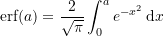with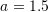• Use Sympy’s built-in numerical integration “integrate” function to calculate an approximation to the true value of the integral. Consider this to be the true value.
• Plot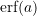for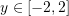• Using the midpoint rectangle rule, provide a table with the following columns: n,h,I_2,|E| with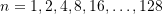• Using the trapezoidal rule, provide a table with the following columns: n,h,I_T,|E| with• Using Simpson’s 1/3 rule, provide a table with the following columns: n,h,I_S1,|E| with• Using Simpson’s 3/8 rule, provide a table with the following columns: n,h,I_S2,|E| with• Comment on the accuracy obtained using each method